• 先给大家介绍下python中random模块 random与numpy.random对比： 1、random.random():生成[0,1)之间的随机浮点数； numpy.random.random():生成[0,1)之间的随机浮点数； numpy.random.random(size=(2,2)),生产一个2维...
• Python中的random模块用于生成随机数。下面介绍一下random模块中最常用的几个函数。 random.random random.random()用于生成一个0到1的随机符点数: 0 <= n < 1> b，则生成的随机数n: a <= n <= b。如果 a ...
• 主要介绍了Python实现简单生成验证码功能,结合实例形式分析了Python基于random模块生成随机字符串的相关操作技巧,需要的朋友可以参考下
• 主要介绍了Python使用random模块生成随机数操作,结合具体实例形式详细分析了random模块生成随机数的各种常用技巧与相关操作注意事项,需要的朋友可以参考下
• 常用的 random 模块方法 import random # random.random()用于生成一个 0 到 1 的随机浮点数： 0 <= n < 1.0 print(random.random()) # 0.18246795790915304 # random.randint(a, b)，用于生成一个指定范围内...
• 主要介绍了Python内置random模块生成随机数的方法,本文给大家介绍的非常详细，具有一定的参考借鉴价值，需要的朋友可以参考下
• random 模块是Python自带的模块，除了生成最简单的随机数以外，还有很多功能。 random.random() 用来生成一个0~1之间的随机浮点数，范围[0,10 >>> import random >>> random.random() 0.5038461831828231 random...
• random模块 用于生成伪随机数 源码位置： Lib/random.py（看看就好，千万别随便修改） 真正意义上的随机数（或者随机事件）在某次产生过程中是按照实验过程中表现的分布概率随机产生的，其结果是不可预测的，是不...
• 主要介绍了Python3 Random模块代码详解，具有一定参考价值，需要的朋友可以了解下。
• 主要介绍了Python基础random模块随机数的生成，文中通过示例代码介绍的非常详细，对大家的学习或者工作具有一定的参考学习价值，需要的朋友们下面随着小编来一起学习学习吧
• ## Python random模块常用方法的使用

千次阅读 多人点赞 2019-07-21 23:36:54
Python random模块常用方法的使用 Python 的random模块包含许多随机数生成器。 random是Python标准库之一，直接导入即可使用。本文介绍random中常用方法的用法。 一、生成随机的整数 # coding=utf-8 import ...
Python random模块常用方法的使用
Python 的random模块包含许多随机数生成器。
random是Python标准库之一，直接导入即可使用。本文介绍random中常用方法的用法。
一、生成随机的整数
# coding=utf-8
import random

print(random.randint(1, 5))
print(random.randrange(0, 51, 5))
运行结果：
3
40
randint(start, end)会返回一个start到end之间的整数，这里是左闭右闭区间。也就是说可能会返回end值，在Python中，这个是一个比较特殊的地方，一般来说都是左闭右开的区间。
randrange(start, end, step)返回一个range(start, end, step)生成的列表中的随机一个值。这里是左闭右开区间，如果上面代码的51如果换成50，则不可能返回50。
每次运行的结果是不同的，因为是随机返回其中一个。
二、生成随机的小数
print(random.random())
print(random.uniform(2, 5))
运行结果：
0.49541524802465897
2.948656863619216
random()返回0到1之间的任意一个浮点数。
uniform(start, end)返回start到end之间的任意一个浮点数，其实就是将random()的结果乘(end-start)，代码就是这么实现的。
三、从字符串、列表、元组中随机返回一个值
choice_str = 'python'
print(random.choice(choice_str))
choice_list = [i for i in range(1, 6)]
print("choice_list: ", choice_list)
print(random.choice(choice_list))
choice_tuple = (10, 20, 30, 40, 50)
print(random.choice(choice_tuple))
运行结果：
h
choice_list:  [1, 2, 3, 4, 5]
5
20
choice()返回可迭代对象中的一个值。可以是列表、元组、字符串，不能是字典和集合。
四、从字符串、列表、元组中随机返回一次或多次，且设置权重
choice_list = [i for i in range(1, 6)]
print("choice_list: ", choice_list)
print(random.choices(choice_list))
choice_tuple = (10, 20, 30, 40, 50)
print(random.choices(choice_tuple, k=2))
choice_str = 'python'
print(random.choices(choice_str, weights=[0.5, 0, 0.5, 0, 0, 0], k=7))
print(random.choices(choice_str, cum_weights=[0, 0.5, 0.5, 1, 1, 1], k=7))
运行结果：
choice_list:  [1, 2, 3, 4, 5]

[20, 50]
['t', 'p', 't', 'p', 'p', 't', 't']
['h', 'y', 'h', 'h', 'h', 'h', 'y']
choices(population, weights=None, cum_weights=None, k=1)从可迭代对象中返回一次或多次值，返回结果是一个列表。
weights是每一个值对应的权重列表，默认是None，所有元素权重相等。传入权重列表时，列表长度必须与可迭代对象的长度相等，值与权重按索引一一对应。传入的值可以是任何数字，只要能对比出不同值之间的权重大小就可以，系统会按权重大小来计算返回每个值的可能性，如上面的例子中只会返回“python”中的p和t，因为其他字符的权重为0。
cum_weights是每一个值对应的累计权重列表，默认是None，所有元素权重相等。传入权重列表时，列表长度必须与可迭代对象的长度相等。权重是累计的，每一个位置的累计权重是前面所有元素的权重之和加上当前位置元素的权重，也就是说这个列表后面的值不可能小于前面的值。系统会根据累计权重值计算出每个元素的权重，然后计算返回每个值的可能性，如上面的例子中只会返回“python”中的y和h,因为其他字符的权重是0。
注意：weights和cum_weights不能同时有值，即使计算结果一样。
k值是从可迭代对象中获取值的次数，每次只取其中的一个，重复k次，所以，理论上，有可能k次都取到同一个元素。
五、从字符串、列表、元组中随机获取一个或多个值
sample_list = [i for i in range(1, 6)]
print("sample_list: ", sample_list)
print(random.sample(sample_list, 1))
sample_tuple = (10, 20, 30, 40, 50)
print(random.sample(sample_tuple, 2))
sample_str = 'python'
print(random.sample(sample_str, 3))
运行结果：
sample_list:  [1, 2, 3, 4, 5]

[10, 40]
['p', 'h', 'o']
sample(population, k)从可迭代对象中随机返回k个元素，返回一个列表。
k表示返回元素的个数，这个参数没有默认值，必须传值。并且，是一次从可迭代对象中返回k个值，不是分k次，每个元素不会重复被取。此外，k值不可以大于可迭代对象的长度，否则报错，而上面choices中的k可以无限大，只要需要。使用场景不同，要注意区别。
六、随机打乱顺序
cards = ['%s-%s' % (a, b) for a in ['Spade', 'Heart', 'Diamond', 'Club'] for b in
([str(i) for i in range(3, 11)] + [j for j in 'JQKA2'])] + ['Black joker', 'Red joker']
print("Before: ", cards)
random.shuffle(cards)
print("After: ", cards)
运行结果：
Before:  ['Spade-3', 'Spade-4', 'Spade-5', 'Spade-6', 'Spade-7', 'Spade-8', 'Spade-9', 'Spade-10', 'Spade-J', 'Spade-Q', 'Spade-K', 'Spade-A', 'Spade-2', 'Heart-3', 'Heart-4', 'Heart-5', 'Heart-6', 'Heart-7', 'Heart-8', 'Heart-9', 'Heart-10', 'Heart-J', 'Heart-Q', 'Heart-K', 'Heart-A', 'Heart-2', 'Diamond-3', 'Diamond-4', 'Diamond-5', 'Diamond-6', 'Diamond-7', 'Diamond-8', 'Diamond-9', 'Diamond-10', 'Diamond-J', 'Diamond-Q', 'Diamond-K', 'Diamond-A', 'Diamond-2', 'Club-3', 'Club-4', 'Club-5', 'Club-6', 'Club-7', 'Club-8', 'Club-9', 'Club-10', 'Club-J', 'Club-Q', 'Club-K', 'Club-A', 'Club-2', 'Black joker', 'Red joker']

shuffle()将可迭代对象的顺序随机打乱，上面例子中我们模拟了一副扑克牌，然后用shuffle()完成“洗牌”。
注意：shuffle()没有返回值，是直接对可迭代对象进行修改。

random除了上面的方法外，还有一些按数学分布随机生成数据的方法，如正太分布，指数分布等，这里就不继续举例了，其实random源码也不是很多，里面的方法数量是人工可数的，需要的话您可以在Pycharm中按住Ctrl点进去看看。展开全文• 随机数的使用，对应文件StandardLibrary8，fortune，文章：https://blog.csdn.net/yysyangyangyangshan/article/details/85042427
• 目录1. choice() 方法 --- 从非空...博主发现当我们自己不知道 Python 某个模块的用法时，自己又没有相关笔记什么的，一般人很少去官网进行查阅，然后在网上乱找一通，描述的也不详细，非常地浪费时间，故博主决定自己


目录
1. random 模块快用导航2. choice() 方法 --- 从非空序列中返回一个随机元素3. choices() 方法 --- 返回序列的随机元素4. randint() 方法 --- 获取指定范围的随机整数5. random() 方法 --- 获取 0.0~1.0 范围内随机浮点数6. randrange() 方法 --- 获取指定范围的随机整数7. sample() 方法 --- 返回无重复随机数列表8. shuffle() 方法 --- 将指定序列中的元素顺序随机排列9. uniform() 方法 --- 获取指定范围之间的随机浮点数10. seed() 方法 --- 初始化随机数生成器

博主发现当我们自己不知道 Python 某个模块的用法时，自己又没有相关笔记什么的，一般人很少去官网进行查阅，然后在网上乱找一通，描述的也不详细，非常地浪费时间，故博主决定自己将常用模块中常用的方法进行总结，以便后期自己查阅和网上的朋友们查找学习。今天开始总结我们的第一个常用模块：random 模块。

random 模块用于实现各种分布的伪随机数生成器，可以根据不同的实数分布来随机生成值，如随机生成指定范围的整数、浮点数、序列等。该模块中的多数函数都取决于 random()函数 ，该函数通过 Mersenne Twister 作为核心生成器在 [0.0, 1.0) 区间范围内均匀生成随机浮点数。
1. random 模块快用导航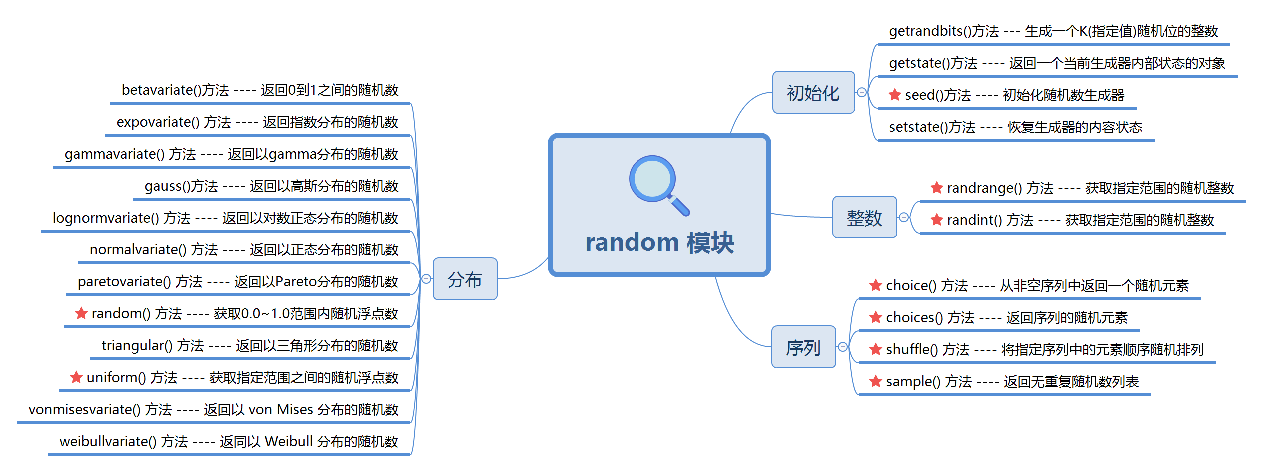2. choice() 方法 — 从非空序列中返回一个随机元素
choice() 方法用于从非空序列中返回一个随机元素。语法格式如下：
def choice(self, seq):
# 参数说明: seq: 表示需要随机抽取的序列。
# 返回值: 从非空序列中返回一个随机元素。

使用 choice() 方法在指定的序列中随机获取元素，代码如下：
import random  # 导入随机数模块

number_list = [6, 7, 8, 9, 10]  # 数值列表
str_tuple = ('5', '2', '0')  # 字符元组
print(f"列表随机元素为: {random.choice(number_list)}")
print(f"元组随机元素为: {random.choice(str_tuple)}")

程序运行结果如下：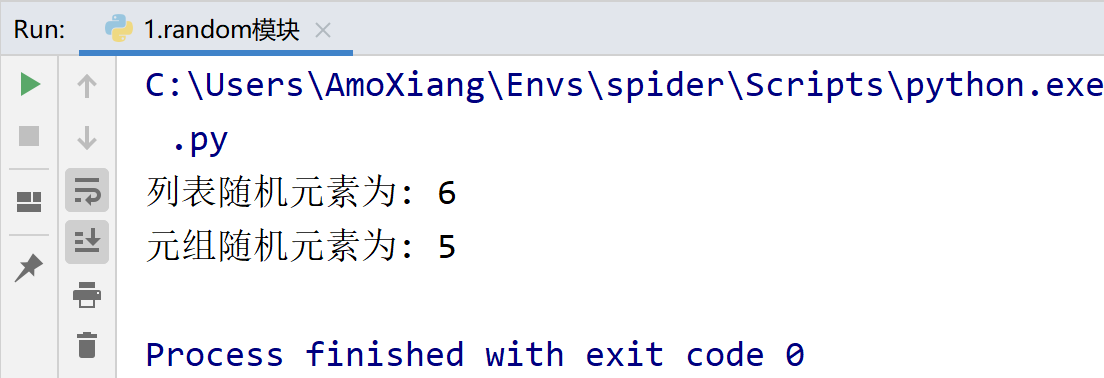3. choices() 方法 — 返回序列的随机元素
choices() 方法是 Python3.6 版本中新增的方法，是 choice() 方法的升级版。语法格式如下：
def choices(self, population, weights=None, *, cum_weights=None, k=1)
参数说明: population: 表示集群中随机抽取 K 个元素。
weights: 表示相对权重列表。
cum_weights: 表示累计权重，该参数与 weights 参数不能同时存在。
返回值: 返回序列的随机元素。

使用 choices() 方法获取指定序列中的随机元素，代码如下：
import random  # 导入随机数模块

print(random.choices(['Amo', 'Jerry', 'Ben']))  # 随机输出列表中的元素
print(random.choices(['Amo', 'Jerry', 'Ben'], k=2))  # 随机输出列表中的元素
# 比如 weights=[1,2,3,4,5], 那么第一个成员的概率就是P=1/(1+2+3+4+5)=1/15
print(random.choices(['Amo', 'Jerry', 'Ben', "Sam", "Paul"], weights=[0, 0, 0, 0, 1]))  # 随机输出列表中的元素

程序运行结果如下：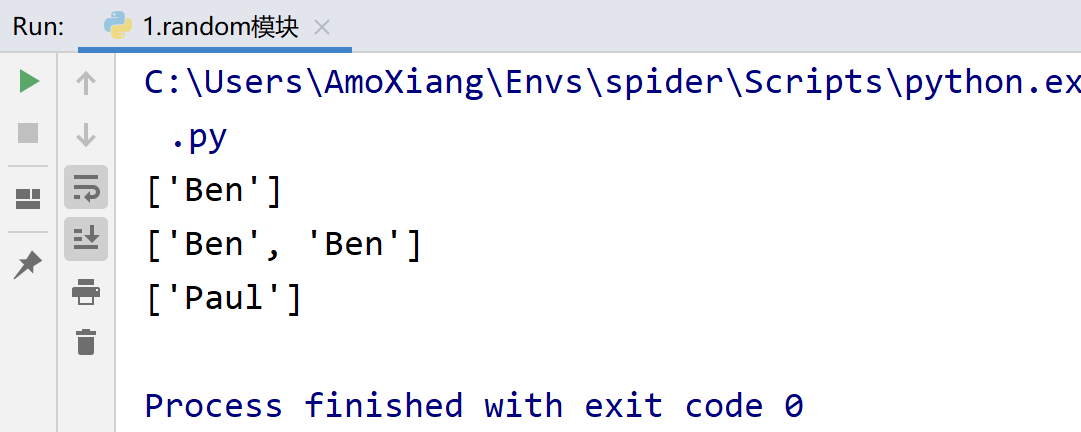4. randint() 方法 — 获取指定范围的随机整数
randint() 方法用于获取指定范围的随机整数。语法格式如下：
def randint(self, a, b)，参数说明如下：
a: 表示随机整数范围的起始值。
b: 表示随机整数范围的结束值，随机出现的整数包含 b 参数。
返回值: 返回指定范围的随机整数。

使用 randint() 方法获取指定范围的随机整数，代码如下：
import random  # 导入随机数模块

print(random.randint(0, 2))  # 打印随机整数

程序运行结果如下：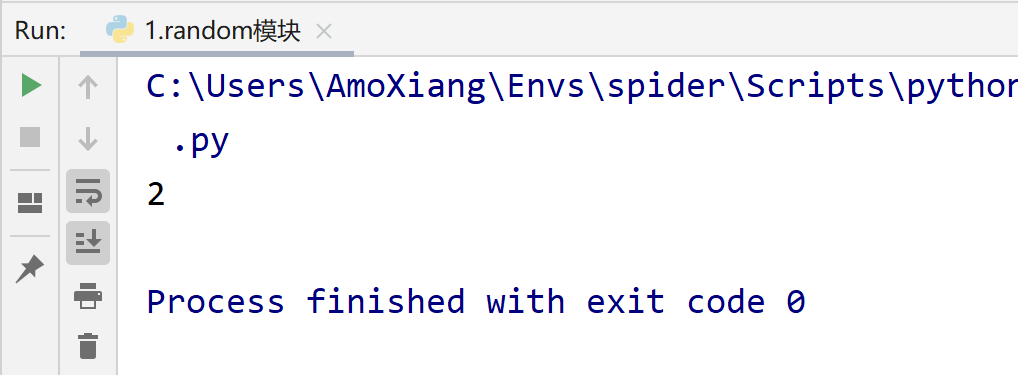5. random() 方法 — 获取 0.0~1.0 范围内随机浮点数
random() 方法用于获取 0.0~1.0 范围内随机浮点数。语法格式如下：
def random(self) 参数说明:
返回值: 返回 0.0~1.0 之间的随机浮点数。

使用 random() 方法获取随机浮点数，代码如下：
import random  # 导入随机数模块

print(random.random())  # 打印0.0至1.0之间的随机浮点数

程序运行结果如下：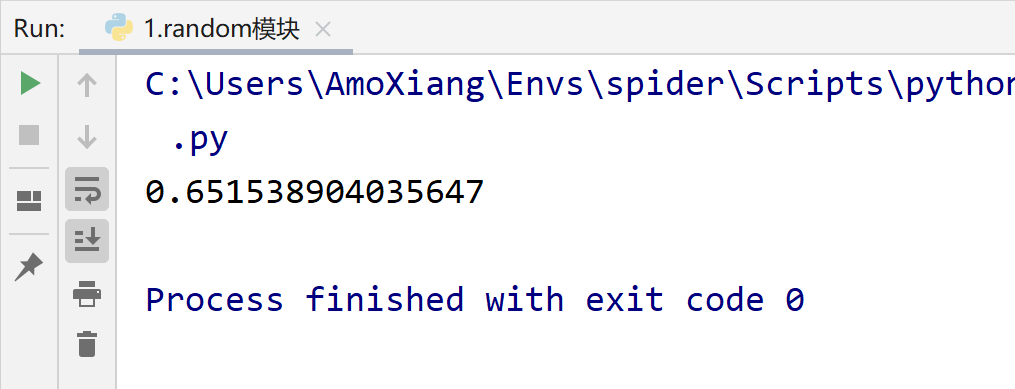6. randrange() 方法 — 获取指定范围的随机整数
randrange() 方法用于获取指定范围的随机整数。语法格式如下：
def randrange(self, start, stop=None, step=1, _int=int)，参数说明:
stop: 在没有指定 start 参数时表示 0 至 stop 之间的一个随机整数，其中不包含 stop 指定的值。
start: 表示随机范围的起始值。指定该参数后，stop 参数将作为随机范围的结束值。
step: 表示步长。
返回值: 返回指定范围的随机整数。

使用 randrange() 方法获取指定范围的随机整数，代码如下：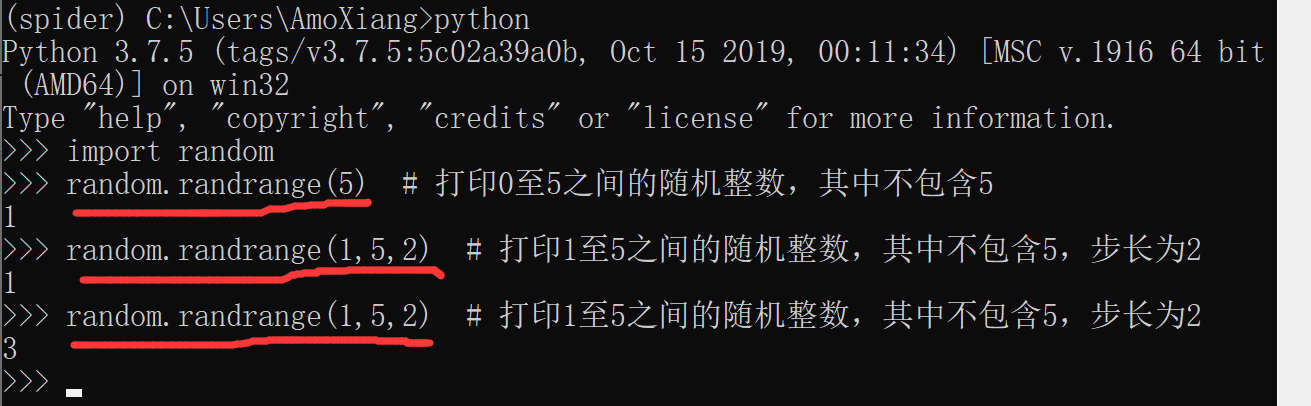在获取第二个随机整数时，由于步长值为2，所以随机整数将在 1 和 3 之间随机出现。
7. sample() 方法 — 返回无重复随机数列表
sample() 方法用于从序列中随机抽取指定数量且不重复的元素所形成的新序列，可处理不可变序列。语法格式如下：
def sample(self, population, k) 参数说明:
population: 表示需要抽取的可变序列或不可变序列。
k: 表示抽取元素的个数。
返回值: 返回在指定序列中抽取指定数量且不重复元素所形成的新序列。

使用 sample() 方法从指定序列中随机抽取指定数量且不重复的元素形成的新序列，代码如下：
import random  # 导入随机数模块

number_list = [1, 2, 3, 4, 5]  # 数值列表
name_tuple = ("Amo", "Jerry", "Ben", "Jason", "Paul")  # 字符元组
print(f"随机抽取可变序列: {random.sample(number_list, 2)}")
print(f"随机抽取不可变序列: {random.sample(name_tuple, 3)}")

程序运行结果如下：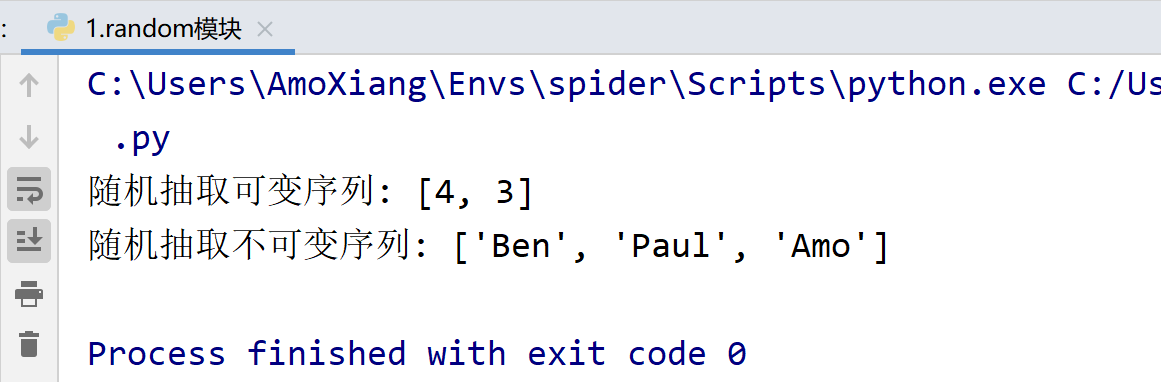8. shuffle() 方法 — 将指定序列中的元素顺序随机排列
shuffle() 方法用于将指定序列中的元素顺序随机排列，只针对可变序列。语法格式如下：
def shuffle(self, x, random=None) 参数说明:
x: 表示需要随机排列元素的序列。
random: 可选参数，返回 [0.0,1.0)中的随机浮点数，默认情况下，该参数为 random() 方法

使用 shuffle() 方法将指定序列中的元素顺序随机排列，代码如下：
import random  # 导入随机数模块

number_list = [1,2,3,4,5,6]  # 数值列表
print(f"原列表顺序为: {number_list}")
random.shuffle(number_list)  # 将列表元素随机排列
print(f"随机排列后的列表: {number_list}")

程序运行结果如下：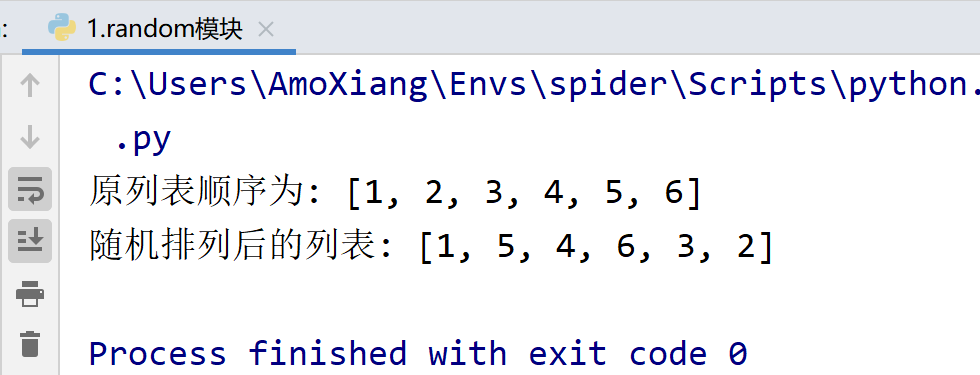9. uniform() 方法 — 获取指定范围之间的随机浮点数
uniform() 方法用于获取指定范围之间的随机浮点数。语法格式如下：
def uniform(self, a, b) 参数说明:
a: 表示指定随机范围的起始值，该值可能会出现在结果中。
b: 表示指定随机范围的结束值，该值可能会出现在结果中。
返回值: 返回一个 a~b 之间随机出现的浮点数。

使用 uniform() 方法获取指定范围之间的随机浮点数，代码如下：
import random  # 导入随机数模块

print(random.uniform(1.0, 5.0))  # 指定参数为浮点数
print(random.uniform(1, 5))  # 指定参数为整数
print(random.uniform(5.0, 1.0))  # 参数a大于参数b

程序运行结果如下：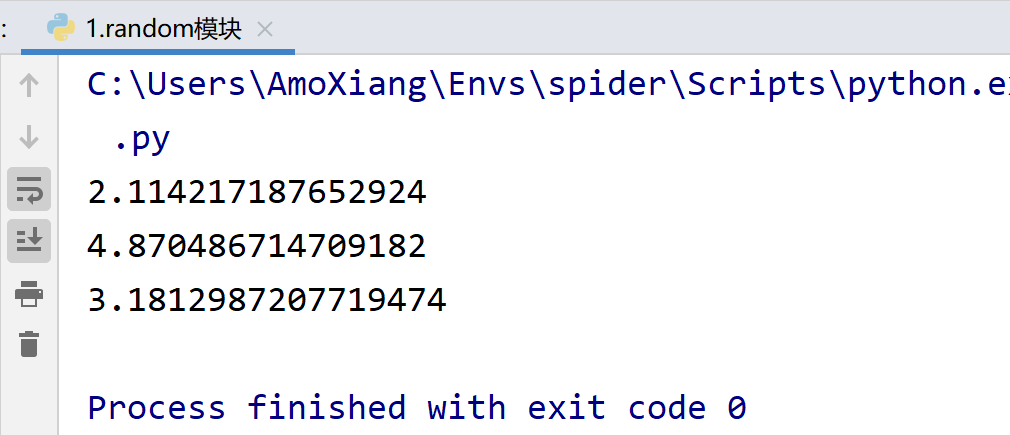10. seed() 方法 — 初始化随机数生成器
seed() 方法用于初始化随机数生成器，可以在调用其他方法之前使用，以实现随机相同的数值。语法格式如下：
def seed(self, a=None, version=2): 参数说明:
a: 表示需要指定的种子，如果未提供 a 或者 a=None，则使用系统时间作为种子。种子相同时即可随机生成相同的数值。
version: 默认值为2，如果参数 a 为整数将不计较参数 version 对应的值是否相同，如果参数 a 为字符类型，此时需要参数 version 对应的值相同。

使用 seed() 方法，通过设置相同的种子数随机生成相同数值，代码如下：
import random  # 导入随机数模块

random.seed()  # 默认种子
print(f"默认种子: {random.random()}")
random.seed()  # 默认种子2
print(f"默认种子2: {random.random()}")
random.seed(a=1)  # 整数种子
print(f"整数种子: {random.random()}")
random.seed(a=1)  # 整数种子2
print(f"整数种子2: {random.random()}")
random.seed(a='1', version=1)  # 字符种子
print(f"字符种子: {random.random()}")
random.seed(a='1', version=1)  # 字符种子2
print(f"字符种子2: {random.random()}")

程序运行结果如下：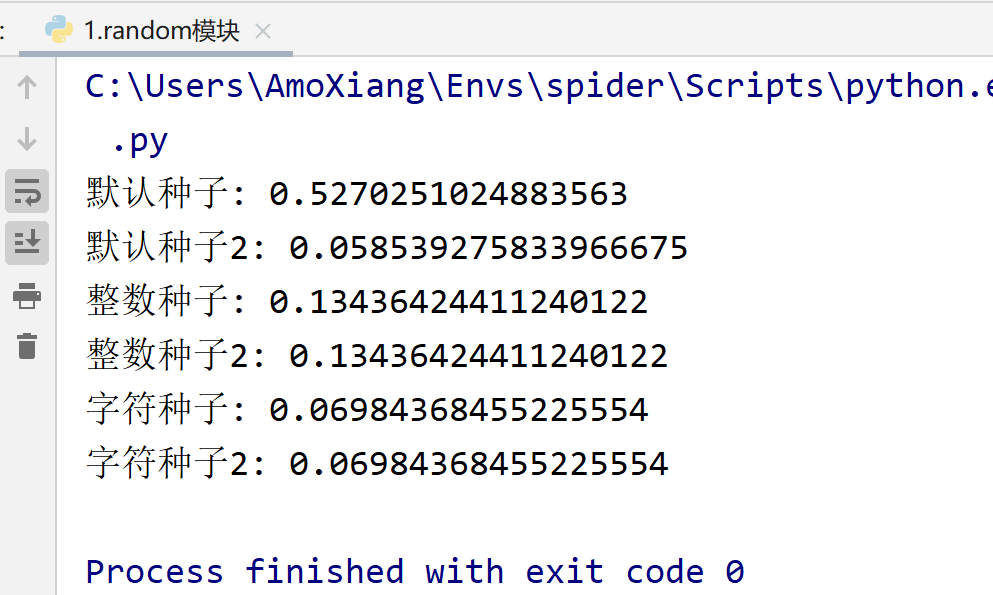展开全文python 列表
• Python 中的的random模块(随机数模块) (1) 取随机小数 random.random() ,随机取一个( 0-1)之间的随机小数 random.uniform(a,b) ,随机取一个(a-b)之间的随机小数 import random print(random.random()) print(random....
• Python 的random模块包含许多随机数生成器。 random是Python标准库之一，直接导入即可使用。本文介绍random中常用方法的用法。 一、生成随机的整数 # coding=utf-8 import random print(random.randint(1, 5)) ...
• random模块 random模块提供了一些生成随机数的函数，一些介绍为了简单省略random. random()：返回在范围大于等于0.0，且小于1.0内的随机浮点数 randrange(stop)：返回大于等于0，且小于 randrange(start, stop[, ...
• random模块用于生成随机数，下面看看模块中一些常用函数的用法： from numpy import random numpy.random.uniform(low=0.0, high=1.0, size=None) 生出size个符合均分布的浮点数，取值范围为[low, high)，默认取值...
• 本章节介绍random模块，分享给刚学python的小伙伴，一起学习，共同进步 random模块的基本操作 import random # 0到1的随机浮点数 print(random.random()) # 随机输出1-10之间的任意一个整数，包含1和10 print(random...
• 主要介绍了Python3.5内置模块之random模块用法,结合实例形式分析了Python3.5 random模块生成随机数与随机字符串相关操作技巧,需要的朋友可以参考下
• 本文实例讲述了Python中random模块用法。分享给大家供大家参考。具体如下： import random x = random.randint(1,4); y = random.choice(['appale','banana','cherry','durian']); print(x,y); 运行结果如下： (2,...
• ## random模块

千次阅读 2016-08-27 19:35:55
random.random作用：用于生成一个0到1的随机符点数: 0 例：>>> import random >>> random.random() 0.533307237462 >>> random.random() 0.366637049337random.uniform函数原型： random.uniform(a, b)作用：用于...
random.random
作用：用于生成一个0到1的随机符点数: 0 <= n < 1.0
例：
>>> import random
>>> random.random()
0.533307237462
>>> random.random()
0.366637049337

random.uniform
函数原型： random.uniform(a, b)
作用：用于生成一个指定范围内的随机符点数，两个参数其中一个是上限，一个是下限。如果a > b，则生成的随机数n: a <= n <= b。如果 a
>>> import random
>>> random.uniform(10,30)
14.415002694
>>> random.uniform(150,300)
250.497992528
>>>


random.randint
函数原型： random.randint(a, b)
作用：用于生成一个指定范围内的整数。其中参数a是下限，参数b是上限，生成的随机数n: a <= n <= b
例：
>>> import random
>>> random.randint(10,20)
11
>>> random.randint(10,20)
15
>>>

random.randrange
函数原型： random.randrange([start], stop[, step])
作用：从指定范围内，按指定基数递增的集合中 获取一个随机数。random.randrange(10, 100, 2)，结果相当于从[10, 12, 14, 16, … 96, 98]序列中获取一个随机数。random.randrange(10, 100, 2)在结果上与 random.choice(range(10, 100, 2) 等效。
例：
>>> import random
>>> random.randrange(10,50,3)
19
>>> random.randrange(10,50,3)
22
>>>


random.choice
函数原型： random.choice(sequence)。
参数说明：参数sequence表示一个有序类型。list, tuple, 字符串等都属于sequence。
作用：从序列中获取一个随机元素。
例：
>>> import random
str_list = ["JGood", "is", "a", "handsome", "boy"]
>>> name_list = ["JGood", "is", "a", "handsome", "boy"]
>>> random.choice(str_list)
'boy'
>>> random.choice(str_list)
'a'
>>> random.choice(str_list)
'is'
>>> random.choice(str_list)
'a'
>>> random.choice(str_list)
'boy'
>>>


random.shuffle
函数原型： random.shuffle(x[, random])
作用：用于将一个列表中的元素打乱。
例：
>>> import random
>>> p = ["Python", "is", "powerful", "simple", "and so on..."]
>>> random.shuffle(p)
>>> p
['powerful', 'simple', 'and so on...', 'Python', 'is']
>>> 

random.sample
函数原型： random.sample(sequence, k)
作用：从指定序列中随机获取指定长度的片断。sample函数不会修改原有序列。
例：
>>> import random
>>> list = [1, 2, 3, 4, 5, 6, 7, 8, 9, 10]
>>> slice = random.sample(list, 5)  #从list中随机获取5个元素，作为一个片断返回
>>> slice
[3, 8, 5, 10, 4]
>>>

展开全文• ## random模块详解

千次阅读 2019-07-02 22:17:40
random模块 导入模块 import random random.randrange() 返回[1,100)范围内的随机数整数，不包括100 >>> random.randrange(1,100) 68 >>> random.randrange(1,100,3) 16 注意：第三个参数为步长...
random模块
导入模块 import random
random.randrange()
返回[1,100)范围内的随机数整数，不包括100
>>> random.randrange(1,100)
68
>>> random.randrange(1,100,3)
16

注意：第三个参数为步长，步长为三时，只能返回该范围内特定的数 比如该例子中[1,4,7,…,97]
random.randrange()
返回[1,100]范围内的随机数整数，包括100,第三个参数同random.randrange()用法一样
>>> random.randint(1,100)
17

random.random()
返回[0, 1)范围内随机浮点数,不包括1
>>> random.random()
0.41385723239524297

random.choice()
在给定容器中随机选择一个元素
>>> random.choice("abcde")
'e'
>>> random.choice([1,2,3])
3
>>> random.choice({1:"a",2:"b"})
'a'

random.sample()
在给定容器中选择特定元素
>>> random.sample("abcde",2)
['e', 'b']

random.shuffle()
随机打乱传入的容器(容器必须是可变对象)
>>> l = [1,2,3,4]
>>> random.shuffle(l)
>>> l
[1, 3, 2, 4]

相应案例
制作图片验证码
前提知识： string模块内置常量
ascii_lowercase = 'abcdefghijklmnopqrstuvwxyz'     小写字母
ascii_uppercase = 'ABCDEFGHIJKLMNOPQRSTUVWXYZ'     大写字母
ascii_letters = ascii_lowercase + ascii_uppercase  大写字母+小写字母
digits = '0123456789'                              数字
hexdigits = digits + 'abcdef' + 'ABCDEF'           十六进制数
octdigits = '01234567'                             十进制数
printable = digits + ascii_letters + punctuation + whitespace 可打印数

具体代码: 使用pillow模块制作验证码图片
展开全文• python中的numpy.random模块提供了常用的随机数生成方法，下面简要总结。 按均匀分布生成随机数 rand 功能 按照均匀分布，在[0,1)内生成随机数。 接口 Docstring: rand(d0, d1, ..., dn) Random values in a given ...
• ## python random模块

千次阅读 2019-06-21 15:21:30
描述: ...注意：random()是不能直接访问的，需要导入 random 模块，然后通过 random 静态对象调用该方法。 返回值: 返回随机生成的一个实数，它在[0,1)范围内。 实例: #!/usr/bin/env python # -*...
• 本节我们学习random模块，此模块主要用于生成随机数。下面介绍一下random模块中最常见的几个函数。 random.random()函数 random()函数用于生成一个0到1之间（0<= n < 1.0）的浮点数。你不能直接使用random()...python...